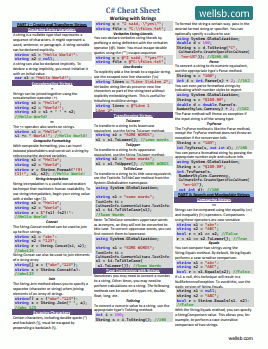# C# Initialize Array

## HOW TO INITIALIZE AN ARRAY IN C#

by

The Array data structure is often better than individually declared variables. This tutorial will cover when to use arrays and how to initialize an array in C#.

## Why use an Array?

Often, you will need to work with several related variables of the same data type. An array allows you to declare multiple variables and treat them as part of a single group. This makes data processing and variable manipulation much easier to code and more efficient to execute.

Suppose you have several related Integer variables and you want to know which variable holds a certain value. If you were to use individually declared variables, you might set up a series of if... else if... statements in order to identify which variable contains the value you are interested in. This is a very poor approach from a programming perspective - it is painfully laborious to code, and takes more clock cycles to execute. With arrays, there is a better way!

If you were to declare each of these integers as part of an array structure, you would be able to loop through the array and check for the value you are interested in. You might use a For Loop in this scenario to find out which variable contains the specific value.

## How to Declare an Array in C#

To declare an array in C#, you will use the syntax:

``````dataType[] arrayName = new dataType[arrayLength];
``````

Create a New .NET Core Console Project in Visual Studio. Add the following line of code inside the `static void Main()` code block:

``````int[] numbers = new int;
``````

With this line, we have declared an array of Integer values. Our array has a length of 5; this means the array contains 5 integers, so it can hold 5 integer values. We can assign values to each integer in our array.

``````int[] numbers = new int;

numbers = 2;
numbers = 4;
numbers = 8;
numbers = 16;
numbers = 32;
``````

We reference individual items in our array structure by the item's index. Index counting starts with the number 0, so our five items will have indices numbered 0-4. numbers is the first item in the array, numbers is the second item, and so forth.

## A Better Way to Initialize Array Values

There is a simpler way to initialize an array in C#.

``````int[] numbers = new int[] { 2, 4, 8, 16, 32 };
``````

Using this syntax, we have both declared our array and set initial values for the elements in the array with a single line of code. This yields the same result as Lines 9-15 in our previous example. In both cases, our program will allocate memory in order to store an array of five integers, and it will assign the same values to each of those integers.

Notice, we did not enter a length for this array. By including initial values inside the curly braces, the compiler will figure out the array's size based on the number of elements that are initialized.

We can use the same syntax to create an array of other datatypes, like strings:

``````string[] names = new string[] { "Brad", "Brian", "Bob", "Bill" };
``````

## Accessing Values of Array Elements

If you want to read the value of a specific element in your array, you would reference the item with the correct index number.

``````using System;

namespace Arrays
{
class Program
{
static void Main(string[] args)
{
int[] numbers = new int[] { 2, 4, 8, 16, 32 };
Console.WriteLine(numbers);
}
}
``````

For example, if you want to print the value of the third variable in our array of integers, you might use the snippet above and you would expect the value `8` to be printed to the console.

## The Bottom Line

Arrays are very useful data structure in the C# programming language. They allow you to quickly declare and efficiently process related variables of the same data type. In this tutorial, you were introduced to arrays and you learned the benefits of using arrays. We presented the basic syntax for declaring arrays, and showed a simple method for initializing arrays in C#. You also learned how to access the values of elements in your array according to their index number. Finally, we shared a complete example where an array was declared, initial values were set, and the value of a specific element was written to the console.